# GSEB Solutions Class 6 Maths Chapter 4 Basic Geometrical Ideas Ex 4.2

Gujarat Board GSEB Textbook Solutions Class 6 Maths Chapter 4 Basic Geometrical Ideas Ex 4.2 Textbook Questions and Answers.

## Gujarat Board Textbook Solutions Class 6 Maths Chapter 4 Basic Geometrical Ideas Ex 4.2

Question 1.
Classify the following curves as
(i) open or
(ii) closed.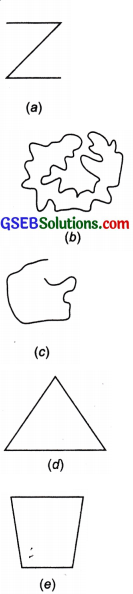Solution:
(a) Open curve
(b) Closed curve
(c) Open curve
(d) Closed curve
(e) Closed curveQuestion 2.
Draw rough diagrams to illustrate the following:
(a) Open curve
(b) Closed curve
Solution: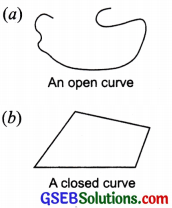Question 3.
Draw any polygon and shade its interior
Solution:
ABCDE is a polygon whose interior is shaded.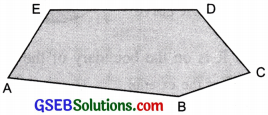Question 4.
Consider the given figure and answer the questions:
(a) Is it a curve?
(b) Is it closed?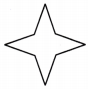Solution:
(a) Yes, it is a curve.
(b) Yes, it is a closed curve.

Question 5.
Illustrate, if possible, each one of the following with a rough diagram:
(a) A closed curve that is not a polygon.
(b) An open curve made up entirely of line segments.
(c) A polygon with two sides.
Solution: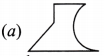is a closed curve but not a polygon because a polygon is made up of line segments only.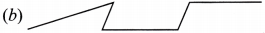(c) A polygon with two sides is not possible.